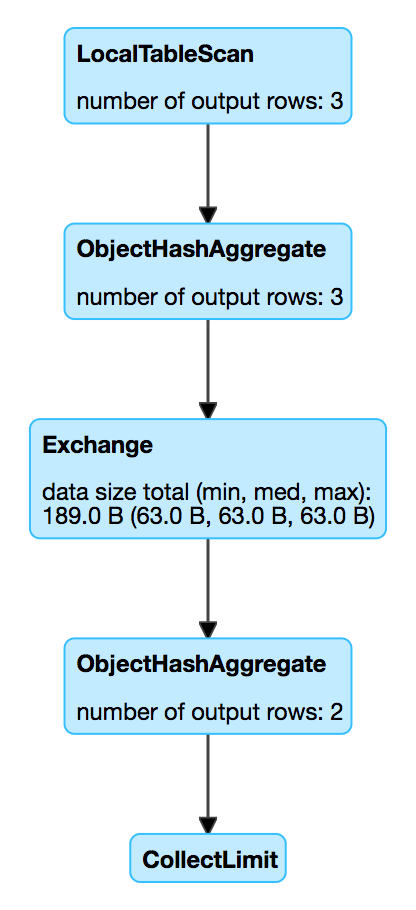## ObjectHashAggregateExec Aggregate Physical Operator

`ObjectHashAggregateExec` is a unary physical operator (i.e. with one child physical operator) that is created (indirectly through AggUtils.createAggregate) when:

• …​FIXME

``````// ObjectHashAggregateExec selected due to:
// 1. spark.sql.execution.useObjectHashAggregateExec internal flag is enabled
scala> val objectHashEnabled = spark.conf.get("spark.sql.execution.useObjectHashAggregateExec")
objectHashEnabled: String = true

// 2. The following data types are used in aggregateBufferAttributes
// BinaryType
// StringType
// ArrayType
// MapType
// ObjectType
// StructType
val dataset = Seq(
(0, Seq.empty[Int]),
(1, Seq(1, 1)),
(2, Seq(2, 2))).toDF("id", "nums")
import org.apache.spark.sql.functions.size
val q = dataset.
groupBy(size(\$"nums") as "group"). // <-- size over array
agg(collect_list("id") as "ids")
scala> q.explain
== Physical Plan ==
ObjectHashAggregate(keys=[size(nums#113)#127], functions=[collect_list(id#112, 0, 0)])
+- Exchange hashpartitioning(size(nums#113)#127, 200)
+- ObjectHashAggregate(keys=[size(nums#113) AS size(nums#113)#127], functions=[partial_collect_list(id#112, 0, 0)])
+- LocalTableScan [id#112, nums#113]

scala> println(q.queryExecution.sparkPlan.numberedTreeString)
00 ObjectHashAggregate(keys=[size(nums#113)#130], functions=[collect_list(id#112, 0, 0)], output=[group#117, ids#122])
01 +- ObjectHashAggregate(keys=[size(nums#113) AS size(nums#113)#130], functions=[partial_collect_list(id#112, 0, 0)], output=[size(nums#113)#130, buf#132])
02    +- LocalTableScan [id#112, nums#113]

// Going low level...watch your steps :)

// copied from HashAggregateExec as it is the preferred aggreate physical operator
// and HashAggregateExec is checked first
// When the check fails, ObjectHashAggregateExec is then checked
import q.queryExecution.optimizedPlan
import org.apache.spark.sql.catalyst.plans.logical.Aggregate
val aggLog = optimizedPlan.asInstanceOf[Aggregate]
import org.apache.spark.sql.catalyst.planning.PhysicalAggregation
import org.apache.spark.sql.catalyst.expressions.aggregate.AggregateExpression
val aggregateExpressions: Seq[AggregateExpression] = PhysicalAggregation.unapply(aggLog).get._2
val aggregateBufferAttributes = aggregateExpressions.
flatMap(_.aggregateFunction.aggBufferAttributes)
import org.apache.spark.sql.execution.aggregate.HashAggregateExec
// that's one of the reasons why ObjectHashAggregateExec was selected
// HashAggregateExec did not meet the requirements
scala> val useHash = HashAggregateExec.supportsAggregate(aggregateBufferAttributes)
useHash: Boolean = true

// collect_list aggregate function uses CollectList TypedImperativeAggregate under the covers
import org.apache.spark.sql.execution.aggregate.ObjectHashAggregateExec
scala> val useObjectHash = ObjectHashAggregateExec.supportsAggregate(aggregateExpressions)
useObjectHash: Boolean = true

00 partial_collect_list(id#112, 0, 0)
01 +- collect_list(id#112, 0, 0)
02    +- id#112: int``````
Table 1. ObjectHashAggregateExec’s Performance Metrics
Key Name (in web UI) Description

`numOutputRows`

number of output rowsFigure 1. ObjectHashAggregateExec in web UI (Details for Query)

### Executing Physical Operator (Generating RDD[InternalRow]) — `doExecute` Method

``doExecute(): RDD[InternalRow]``
 Note `doExecute` is part of SparkPlan Contract to generate the runtime representation of a structured query as a distributed computation over internal binary rows on Apache Spark (i.e. `RDD[InternalRow]`).

`doExecute`…​FIXME

### `supportsAggregate` Method

``supportsAggregate(aggregateExpressions: Seq[AggregateExpression]): Boolean``

`supportsAggregate` is enabled (i.e. returns `true`) if there is at least one TypedImperativeAggregate aggregate function in the input `aggregateExpressions` aggregate expressions.

 Note `supportsAggregate` is used exclusively when `AggUtils` is requested to create an aggregate physical operator given aggregate expressions.

### Creating ObjectHashAggregateExec Instance

`ObjectHashAggregateExec` takes the following when created: